Function Repository Resource:

# FromTensor

Convert TensorContract expressions into an equivalent form using Dot and Tr

Contributed by: Carl Woll
 ResourceFunction["FromTensor"][expr] converts TensorContract expressions in expr into equivalent forms using Dot and Tr.

## Details and Options

Only tensors of rank 1 or 2 are supported.
Transpose operations in the output are represented using Transpose instead of TensorTranspose.
ResourceFunction["FromTensor"] makes no assumptions about the rank of the symbolic tensors involved. Use the Assumptions option or \$Assumptions to define rank information for the involved tensors.
The input and output of ResourceFunction["FromTensor"] will evaluate to the same array when given explicit arrays as input.
For numerical tensors, the output of ResourceFunction["FromTensor"] will evaluate much more quickly than the input TensorContract form.

## Examples

### Basic Examples (4)

Specify the properties of symbolic arrays:

 In:=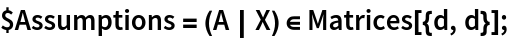Create a TensorContract expression:

 In:=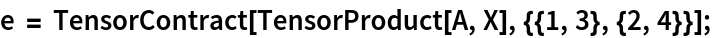Convert to an equivalent form using Tr and Dot:

 In:=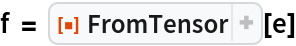Out=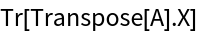Check:

 In:=Out=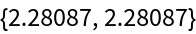### Scope (3)

Create a tensor with disconnected components:

 In:=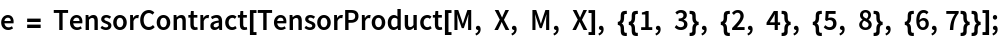Convert to an equivalent form using Tr and Dot:

 In:=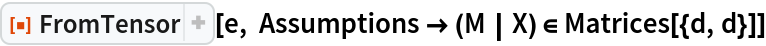Out=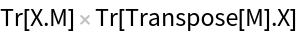Check equivalence of forms:

 In:=Out=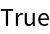### Applications (4)

Tensor forms using Dot and Tr evaluate much more quickly than forms using TensorContract. Consider the following TensorContract expression:

 In:=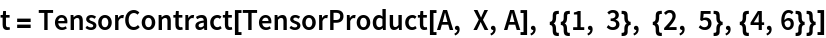Out=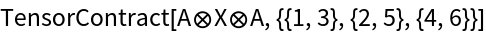The equivalent form using Dot and Tr:

 In:=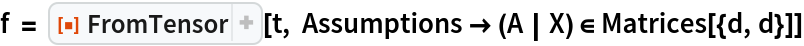Out=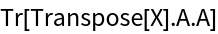Evaluate the two forms of the tensor using numerical arrays as the tensors:

 In:=Out=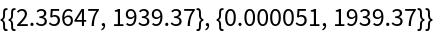The slowness of the TensorContract version can be ameliorated by judicious use of Inactivate and Activate:

 In:=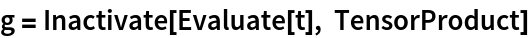Out=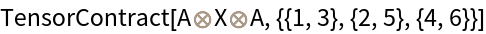In:=Out=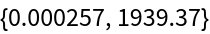Carl Woll

## Version History

• 2.0.0 – 06 November 2020
• 1.0.0 – 16 October 2019